# Limit of Resolution: Rayleigh Criterion

When a point object is imaged using a circular aperture such as the lens or the iris of our eye, the image produced is not a point, rather a diffraction pattern. This is true, mainly when the size of the object is comparable to the wavelength of light.

A circular aperture creates a diffraction pattern of concentric rings that grow dimmer as we move away from the centre. These are known as Airy discs.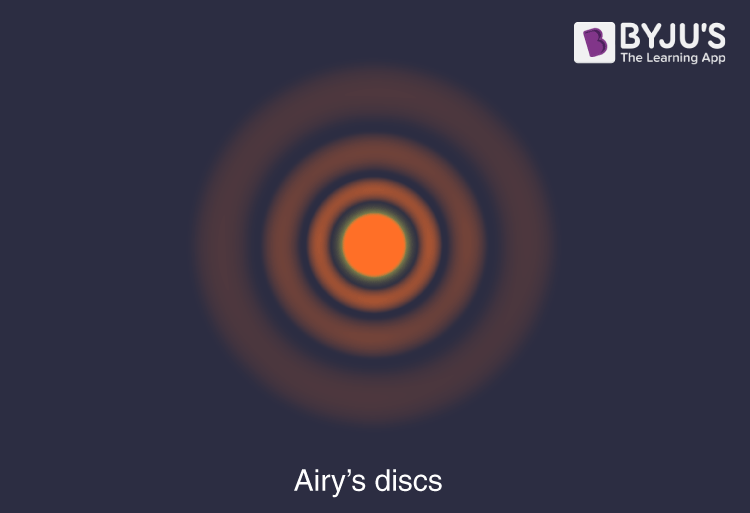Because of the airy discs, point sources close to one another can overlap and produce a blurred image, as shown in the image.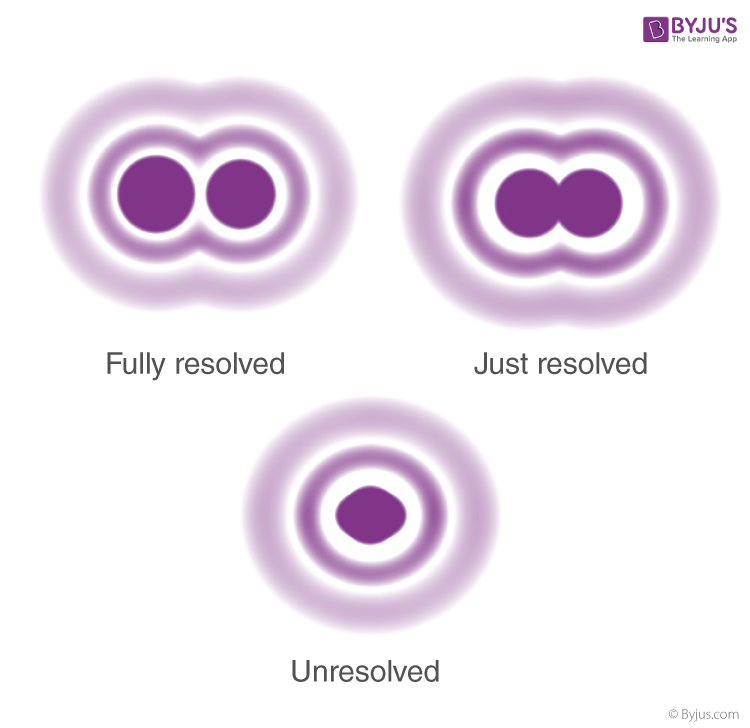To obtain a good image, point sources must be sufficiently far apart that their diffraction patterns do not overlap. To achieve this, the minimum distance between images must be such that the central maximum of the first image lies on the first minimum of the second and vice versa. Such an image is said to be just resolved. This is the famous Rayleigh criterion.

## What is Rayleigh Criterion?

Consider the diffraction pattern for a circular aperture as shown in the figure below.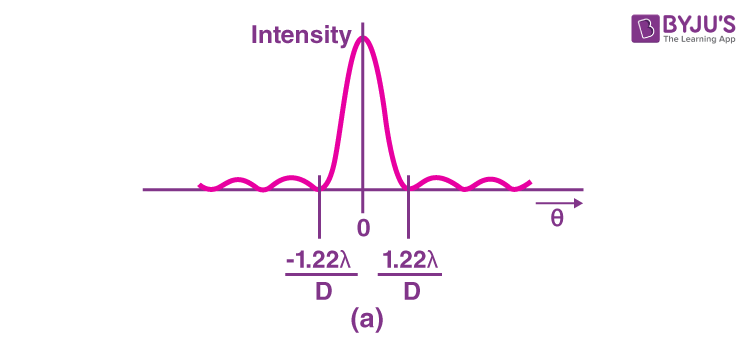It can be shown that, for a circular aperture of diameter D, the first minimum in the diffraction pattern occurs at

$\Theta =1.22\frac{\lambda}{D}$

The accepted criterion for determining the diffraction limit to resolution based on this angle was developed by Lord Rayleigh in the 19th century.

Rayleigh criterion for the diffraction limit to resolution states that two images are just resolvable when the centre of the diffraction pattern of one is directly over the first minimum of the diffraction pattern of the other.”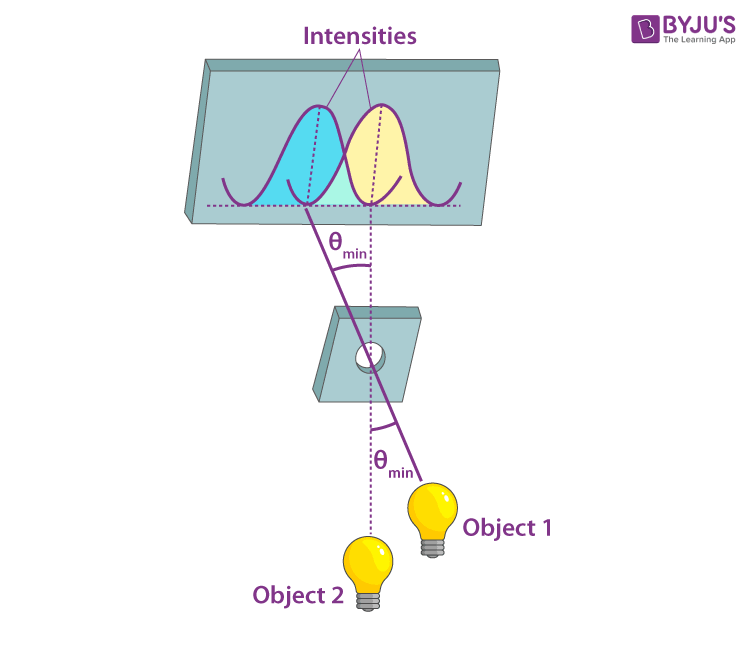In the figure, the first minimum is at an angle

$\Theta =1.22\frac{\lambda}{D}$

So this means that two point objects are just resolvable if they are separated by an angle

$\Theta =1.22\frac{\lambda}{D}$

### Rayleigh Criterion Solved Example

The Hubble Space Telescope’s primary mirror has a diameter of 2.40 m. (a) Determine the angle between two just-resolvable point light sources? Assume the average wavelength of light as 550 nm.

Solution:

The Rayleigh criterion gives the smallest possible angle 𝜃 between point sources.

$\Theta =1.22\frac{\lambda}{D}$

Substituting the values in the above equation, we get:

$\Theta =1.22\frac{\lambda}{D}$

$\Theta =1.22 \frac{220\times 10^{-9}{\,m}}{2.40\,{\,m}}=2.80\times 10^{-7}\,{\,m}$

## Resolving Power of Telescope

In telescopes, objects such as binary stars which are very close to each other, subtend small angles on the telescope. We need large apertures to resolve them. We can make use of Rayleigh’s Criterion to determine the resolving power of the telescope. The angular separation between two objects must be

$\Theta =1.22\frac{\lambda}{D}$

Resolving power is defined as the inverse of the distance or angular separation between two objects which can be resolved through the optical instrument. Therefore,

$Resolving\,Power=\frac{1}{\Delta \Theta }=\frac{d}{1.22\lambda }$

Thus, the higher the diameter d, the better the resolution. Astronomical optical telescopes have mirror diameters as large as 10 m to achieve the best resolution.

### Watch the video and learn how a telescope makes distant objects appear nearer.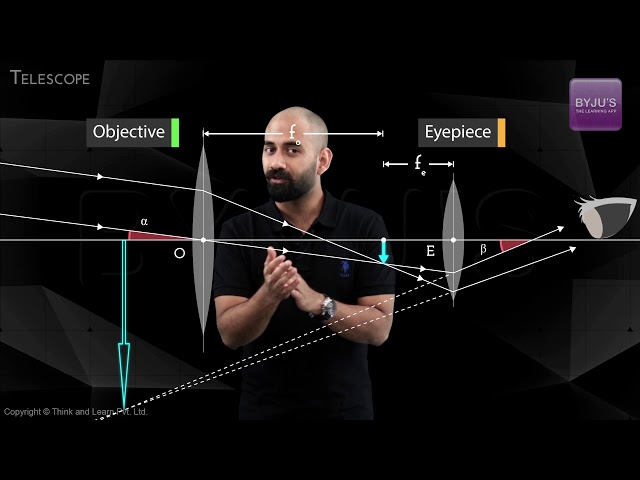Similar Articles:

## Frequently Asked Questions on Limit of Resolution

### What does the Rayleigh criterion attempt to describe?

The Rayleigh criterion describes the minimum separation needed between two light sources to be resolved into distinct objects.

### What is Airy Disc?

A circular aperture creates a diffraction pattern of concentric rings that grow dimmer as we move away from the centre. These are known as Airy discs.

### What do you mean by resolving power?

Resolving power is defined as the inverse of the distance or angular separation between two objects which can be resolved through the optical instrument.

### What does Rayleigh’s criterion state?

“Rayleigh criterion for the diffraction limit to resolution states that two images are just resolvable when the centre of the diffraction pattern of one is directly over the first minimum of the diffraction pattern of the other.”

### Who discovered Rayleigh’s criterion?

Lord Rayleigh developed Rayleigh’s criterion in the 19th century.

Stay tuned to BYJU’S and Fall in Love with Learning!